# Optimisation and Numerical Analysis

The Optimisation and Numerical Analysis group at the School of Mathematics has a range of interests in theoretical and combinatorial optimisation, numerical methods for PDEs and integral equations, dynamical systems, numerical linear algebra, and more, with a wide variety of applications.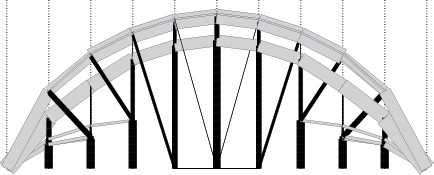In optimisation, the main focus of the research activity of the group is on mathematical theory and methods applicable to managerial decision-making, particularly non-linear, discrete, and multi-criteria optimisation. Research is typically a mixture of theoretical investigation and practical application or industrial collaboration. As an example, the image to the right is of a bridge showing the result of topology optimisation starting from a dense very mesh of potential bars.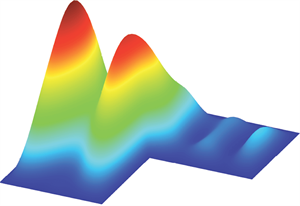Numerical analysis research at Birmingham covers a wide range of areas related to the design, analysis and implementation of robust and accurate numerical algorithms for solving mathematical problems coming from real-life applications. The applications of interest include problems in computational fluid dynamics, plasma dynamics and ecology, electromagnetics, linear elasticity, fuel cell modelling, topology optimization, financial modelling and biological fluid mechanics.

The two subgroups of Optimisation and Numerical Analysis knit together and interact closely, for example organising the biannual IMA Conference on Numerical Linear Algebra and Optimisation since 2007 and the joint Optimisation and Numerical Analysis seminar.

A list of recent grants obtained by the Group can be found on the grants page. Previous PhD students in the Optimisation and Numerical Analysis Group, together with their theses, can be found on the previous PhD students page.

## Head of Optimisation and Numerical Analysis Group

### Dr Alex BespalovAssociate Professor in Numerical Analysis

Dr Bespalov's research interests are in the areas of numerical analysis, scientific computing and uncertainty quantification. His research is primarily focused on numerical methods and adaptive solution algorithms for partial differential and boundary integral equations, with applications to electromagnetics, wave propagation, linear elasticity, and groundwater flow modelling.

### Dr Daniel Jones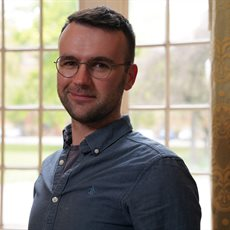Lecturer

Dr Jones has published papers in the area of tropical linear algebra (max-plus algebra) including: special cases of the generalised eigenproblem; links between two-sided systems and the assignment problem and tropical matrix roots. He also has research in fire propagation in urban areas using techniques of: cellular automata, convex analysis and min-plus algebra.

### Professor Michal KočvaraProfessor in Mathematical Optimisation

Professor Kočvara's research interests include non-linear and semidefinite optimisation, optimisation of elastic structures, and optimisation with equilibrium constraints.

### Dr Arnaud LionnetLecturer

Dr Lionnet's research interests span theoretical and numerical analysis of Backward Stochastic Differential Equations (BSDEs), probabilistic numerical methods for PDEs, and systemic risk in financial networks.

### Dr Daniel LoghinAssociate Professor

Dr Loghin has research interests in numerical linear algebra, iterative methods for linear and non-linear systems, finite element methods, and computational fluid dynamics.

### Dr Sándor Zoltán Németh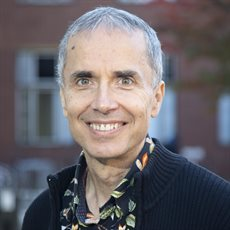Associate Professor

Dr Németh's recent research areas are Optimisation on Manifolds, Convex Optimisation and Equilibrium Systems. He is especially interested in the connections between these areas. Dr Németh's other main topics of interest are Ordered Vector Spaces, Cones and Projection Operators.

### Dr Natalia Petrovskaya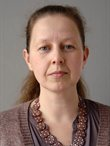Associate Professor in Applied Mathematics

Dr Petrovskaya is interested in mathematical and computational modelling of complex problems in ecology and environment, engineering, and physics. Her research involves theoretical and computational investigation using mathematical models describing real-life phenomena and the design and exploration of new numerical methods required to solve the problem.

### Dr Sergey Sergeev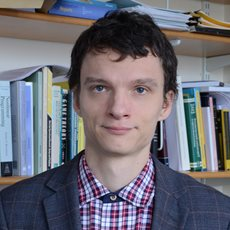Associate Professor in Mathematical Optimisation

Dr Sergeev specialises in max algebra and tropical convexity, along with optimisation in tropical mathematics. He has also worked on public key cryptography based on tropical algebra. Dr Sergeev's current research interests also include Perron-Frobenius theory, bi-level optimisation and its applications, and game theory.

### Dr Xiaocheng ShangAssociate Professor in Mathematical Optimisation and Data Science

Dr Shang's primary research interests lie in the optimal design of numerical methods for stochastic differential equations with a strong emphasis on applications ranging from computational mathematics, statistics, physics, to data science.

### Dr Junqi (Billy) Tang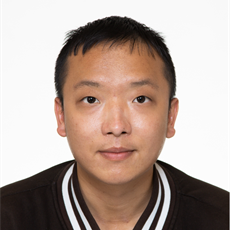Assistant Professor

Dr Tang's research interests include large-scale optimisation, statistical learning theory, and applications in computer vision and medical imaging.

### Dr Yi ZhangAssociate Professor

Dr Zhang's research lies in Markov Decision Processes and Stochastic Games, Markov Chains and Pure Jump Processes, Statistical Decision Theory and Sequential Analysis, and applications to e.g., Impulse Control Problems and Telecommunication.

## PhD Students

Note that not all PhD researchers have requested profiles.